# Cellular textures

Ah, the good old cellular texture generator. Everyone has their own, so here's mine. It's a dumb, brute-force cellular texture generator. It's slow and hard to modify. Nothing fancy like sphere trees are used to accelerate the nearest point search. If you want speed, go read what ryg has to say.

But one good thing about is that everything it outputs is tiling. Even if the output is not square (and non-power-of-two, it will still tile.

By default, the points are put in random places. You can achieve all kinds of nice things if you order them some way. Squares, hexagons, etc. are all possible.

Download cellular.cpp (8 KiB). Compilation requires libpng and zlib. The file is hereby placed into the public domain.

### Examples

Some examples of what this thing can do. Different images can be created by changing the distance equation and changing how the distances are drawn.

Image How to generate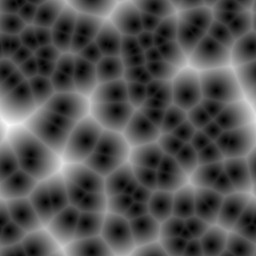Equation: `dist1`
Drawing function: `drawNearest()`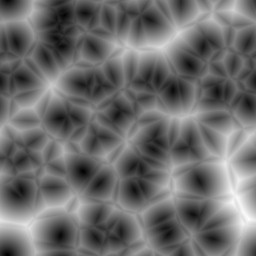Equation: `dist2`
Drawing function: `drawNearest()`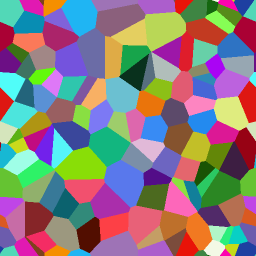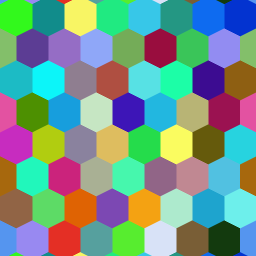Equation: `dist2 - dist1`
Drawing function: `drawSolidVoronoi()`

The second image shows a hexagonal pattern. This is done by putting the points in neat rows, but shifting every second row by half of the distance between the points.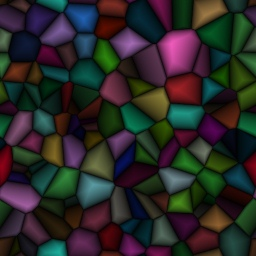Equation: `dist2 - dist1`
Drawing function: `drawFadedVoronoi()`